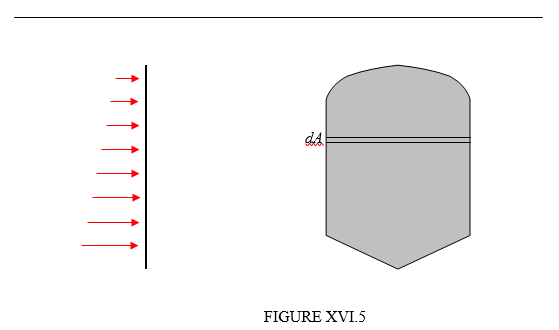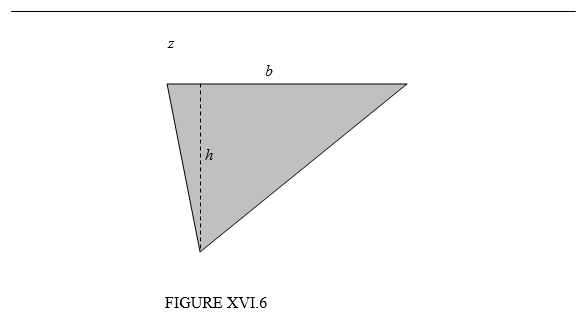$$\require{cancel}$$

16.5: Pressure on a Vertical Surface

•• Contributed by Jeremy Tatum
• Emeritus Professor (Physics & Astronomy) at University of Victoria

Figure XVI.5 shows a vertical surface from the side and face-on. The pressure increases at greater depths. I show a strip of the surface at depth $$z$$.Suppose the area of that strip is $$dA$$. The pressure at depth $$z$$ is $$\rho gz$$, so the force on the strip is $$\rho gzdA$$. The force on the entire area is $$\rho g\int zdA$$, and that, by definition of the centroid (see Chapter 1), is $$\rho g\overline{z}A$$ where $$\overline{z}$$ is the depth of the centroid. The same result will be obtained for an inclined surface.

Therefore:

The total force on a submerged vertical or inclined plane surface is equal to the area of the surface times the depth of the centroid.

Example $$\PageIndex{1}$$

Figure XVI.6 shows a triangular area. The uppermost side of the triangle is parallel to the surface at a depth $$z$$. The depth of the centroid is $$z+\frac{1}{3}h$$, so the pressure at the centroid is $$\rho g\left(z+\frac{1}{3}h\right)$$. The area of the triangle is $$\frac{1}{2}bh$$ so the total force on the triangle is $$\frac{1}{2}\rho gbh\left(z+\frac{1}{3}h\right)$$.